# Eigen values of differential operators, numerical methods

Methods for computing the eigen values and corresponding eigen functions of differential operators. Oscillations of a bounded elastic body are described by the equation(1)

where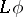is some differential expression. If one seeks solutions of (1) of the formthe following equation foris obtained: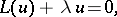(2)

within a bounded domain, under certain homogeneous conditions on the boundary. The values of the parameterfor which there exist non-zero solutions of (2) satisfying the homogeneous boundary conditions are called eigen values, and the corresponding solutions eigen functions. The resulting eigen value problem consists of determining the eigen valuesand the eigen functions corresponding to them (see , ).

The numerical solution of this problem proceeds in three steps:

1) reduction of the problem to a simpler one, for example, to an algebraic (discrete) problem (see , );

2) specification of the precision of this discrete problem;

3) calculation of the eigen values for the discrete problem (see ,  and Linear algebra, numerical methods in).

## Reduction to a discrete problem.

The reduction of problem (2) to a discrete model is usually carried out using the grid method and projection methods. It is natural to require that the basic properties of the original problem, such as the self-adjointness of an operator, be preserved in its discrete analogue.

One method of reduction is the integro-interpolation method. For example, consider the problem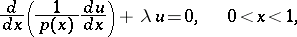(3)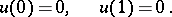(4)

This problem arises, for example, in the study of transverse vibrations of a non-homogeneous string and longitudinal vibrations of a non-homogeneous rod.

On the interval, one introduces the uniform grid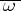with nodes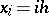,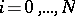,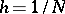. To every node,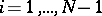, is assigned an elementary domain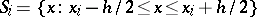. Integration of equation (3) over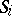yields(5)

Let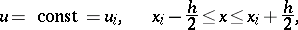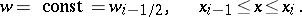Then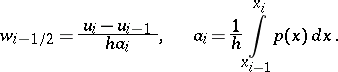(6)

Substituting (6) into (5), one obtains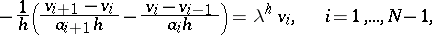whereis the described grid function. The boundary conditions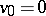,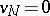reduce to an algebraic eigen value problem: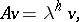(7)

whereis tri-diagonal symmetric matrix of order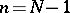.

The variational-difference method of reduction to a discrete problem is used when the eigen value problem can be formulated as a variational one. For example, the eigen values of the problem (3), (4) are the stationary values of the functional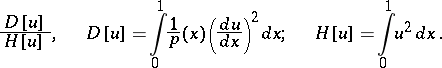By changing the integrals to quadrature sums and the derivatives to difference relations, the discrete analogue of this functional has the form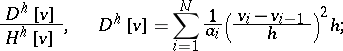where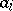is the difference analogue of the coefficient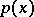, which can be calculated by formula (6). The discrete analogue of the problem (3), (4) is obtained from the necessary condition for an extremum: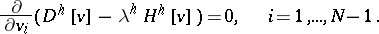Differentiation again leads to the problem (7) (see ).

The projection-difference method of reduction to a discrete problem consists of the following. One chooses a linearly independent system of coordinate functions,, and a linearly independent system of projection functions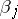,. One seeks approximate eigen functions in the form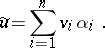The coefficients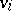and the approximate eigen values are determined by the condition(8)

whereis the scalar product in the Hilbert space. When the coordinate and projection systems coincide, one talks of the Bubnov–Galerkin method. If, in addition, the operator of the differential problem is self-adjoint, the method is called the Raleigh–Ritz method. In particular, for problem (3), (4), if allsatisfy (4), condition (8) takes the form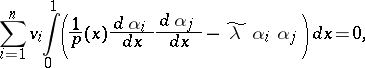(9)In order to simplify the derivation of the algebraic problem, one chooses an almost-orthogonal system of functions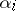.

By taking as coordinate and projection systems functions of the form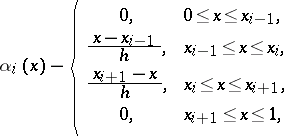where, it follows from (9) that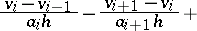where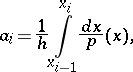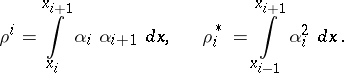Thus, together with the boundary conditions, one obtains a generalized eigen value problem: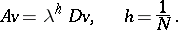Hereandare tri-diagonal symmetric matrices of order.

These methods also yield discrete models of other equations. For example, for a rod: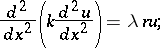for a membrane:and for a plate: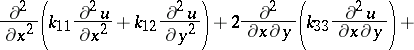The eigen vectors corresponding to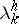satisfy the homogeneous system of algebraic equations: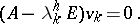The problem of finding all eigen values and eigen vectors of a matrixis called the complete problem of eigen values, and the problem of finding some eigen values ofis called the partial problem of eigen values.

In the case of algebraic systems corresponding to a given problem, the latter problem arises most frequently. The application of traditional solution methods requires a considerable amount of calculation, in view of the poor separation of the eigen values of. In this case it is more effective to use modified gradient methods with spectrally equivalent operators (see - ) and multi-grid methods (see ).